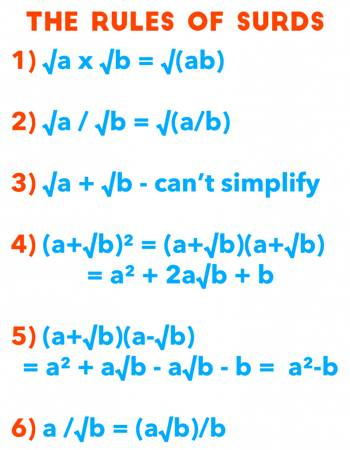Surds are numbers left in root form (√) to express its exact value. It has an infinite number of non-recurring decimals. Therefore, surds are irrational numbers. There are certain rules that we follow to simplify an expression involving surds.

A root of a positive real quantity is called a surd if its value cannot be exactly determined. It is a number that can’t be simplified to remove a square root (or cube root etc). For example, each of the quantities √3, ∛7, ∜19, (16)^2/5 etc. is a surd.

More Examples:

• √2 (square root of 2) can’t be simplified further so it is a surd
• √4 (square root of 4) CAN be simplified to 2, so it is NOT a surdRules of Surds

Some of the important rules of surds are listed below.

1. Every rational number is not a surd.
2. Every irrational number is a surd.
3. A root of a positive real quantity is called a surd if its value cannot he exactly determined.

√9, ∛64, ∜(16/81) etc. are rational numbers but not surds because √9 = 3, ∛64 = 4, ∜(16/81) = 2/3 etc.

1. √a × √a = a ⇒ √5 × √5 = 5
2. The sum and difference of two simple quadratic surds are said to be conjugate surds or complementary surds to each other. Thus, (4√7 + √6) and (4√7 – √6) are surds conjugate to each other.
3. To express in the simplest form, denominator must be rationalized.
4. The method of convening a given surd into a rational number on multiplication by another suitable surd is called rationalization of surds. In this case the multiplying surd is called the rationalizing factor of the given surd and conversely.
5. If a and b are both rationals and √x and √y are both surds and a + √x = b + √y then a = b and x = y
6. If a – √x = b – √y then a = b and x = y.
7. If a + √x = 0, then a = 0 and x = 0.
8. If a – √x = 0, then a = 0 and x = 0.

Information Source;